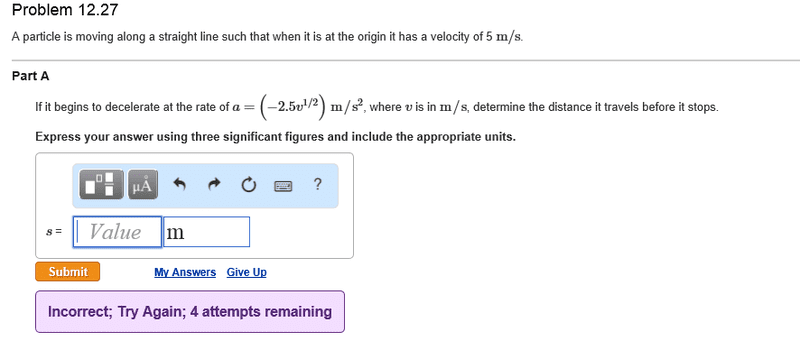# Non uniform acceleration## Homework Statement

A particle is moving along a straight line such that when it is at the origin it has a velocity of 5m/s. If it begins to decelerate at a rate of a=(-2.5v^(1/2)) m/s^2, where v is in m/s, determine the distance it travels before it stops

## The Attempt at a Solution

I integrated the above expression twice with respect to time, to get an expression of s=(2(v^(5/3) t^2))/3+C1+C2. Where C1 ns C2 are constants. I've ended up with more variables than I have the know how to handle. I don't know what to do with the initial velocity. I thought of integrating with respect to v but it didn't make sense. Please help.

SteamKing
Staff Emeritus
Homework Helper

How did you wind up with two constants of integration without one being multiplied by something else?

You know that when x = 0, v = 5 m/s. Did you apply these initial conditions to determine what the constants of integration must be?

RUber
Homework Helper
It stops when v = 0, right?
Graphically, I get an answer of less than 3m.

I would attack it like this:
since acceleration is the derivative of velocity and ##a = -2.5 v^{1/2}##
assume that velocity is of the form ##v(t) = c(t+a)^2##
Then ##v' = 2c(t+a) = -2.5 \sqrt{ c (t+a)^2 } ##.
Using initial information, you should be able to find a suitable solution for c and a, then solve your velocity equation for the time when v = 0.
Last, integrate velocity from 0 to t_final to get total change in position.

Thanks guys. will try it and see what I end up with.

Chestermiller
Mentor
What's wrong with solving the differential equation

$$\frac{dv}{dt}=-2.5v^{\frac{1}{2}}$$

for v as a function of t, subject to the initial condition?

Chet

rcgldr
Homework Helper
I integrated the above expression twice ...
Why twice, you only need to integrate dv once to end up with v, and you're solving for v = 0.

I missed that the question was about distance traveled, not time, so you will need to integrate twice.

What's wrong with solving the differential equation
$$\frac{dv}{dt}=-2.5v^{\frac{1}{2}}$$

So that would be

$$\frac{dv}{v^{\frac{1}{2}}} = -2.5 \ dt$$

Last edited:
Chestermiller
Mentor
Why twice, you only need to integrate dv once to end up with v, and you're solving for v = 0.

So that would be

$$\frac{dv}{-2.5v^{\frac{1}{2}}} = dt$$
I would have had the -2.5 associated with the dt.

Why twice, you only need to integrate dv once to end up with v, and you're solving for v = 0.

So that would be

$$\frac{dv}{v^{\frac{1}{2}}} = -2.5 \ dt$$
I thought I needed to s end up with an expression for position / distance

rcgldr
Homework Helper
I thought I needed to s end up with an expression for position / distance
You're correct. I lost a update to my prior post; and it's fixed now. So yes, you can start with dv/(v^(1/2) = -2.5 dt, and noting that v = ds/dt, this results in some function f(ds/dt) + 5 = t. (5 being the constant of integration, in this case the initial velocity). Again you can separate the equation so ds and the s terms are on one side and dt and the t terms are on the other and integrate again.

Last edited:
ehild
Homework Helper
You can use the distance travelled as independent variable instead of t. dv/dt=(dv/ds)(dv/dt)(ds/dt)=0.5 d(v2)/ds.You need to integrate with respect to s.

Last edited:
rcgldr
Homework Helper
You can use the distance traveled as independent variable instead of t. dv/dt=(dv/ds)(dv/dt)=0.5 d(v2)/ds.You need to integrate with respect to s.
If using chain rule, shouldn't that be dv/dt = (dv/ds)(ds/dt) = v dv/ds ?

ehild
Homework Helper
If using chain rule, shouldn't that be dv/dt = (dv/ds)(ds/dt) = v dv/ds ?

Yes, it was a typo, but the last formula is true. a=dv/dt= 0.5d(v2)/ds.

rcgldr
Homework Helper
Yes, it was a typo, but the last formula is true. a=dv/dt= 0.5d(v2)/ds.
OK, but is it any simpler than just continuing with the chain rule result:

$$v \ \frac{dv}{ds} = -2.5 v^{\frac{1}{2}}$$

$$v^{\frac{1}{2}} \ dv = -2.5 \ ds$$

Which only requires a single integration, while the previously mentioned equation

$$\frac{dv}{v^{\frac{1}{2}}} = -2.5 \ dt$$

requires two integrations.

As for the constant(s) of integration, the problem states that v = 5 m/s at t = 0 and s = 0, and that the initial position is s = 0, which allows you to solve for the constant(s) of integration with either approach. I got the same result for s using both methods.

Last edited:
Ok it took long for me to get back to this thread but I did get it eventually.

•Chestermiller
rcgldr
Homework Helper
If anyone is curious the distance is

$$\frac{4}{3} \ \sqrt{5} \approx 2.981424$$

Last edited:
If anyone is curious the distance is

$$\frac{4}{3} \ \sqrt{5} \approx 2.981424$$
Yep.